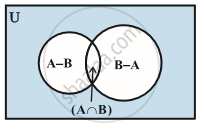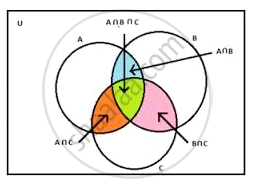# Practical Problems on Union and Intersection of Two Sets

## Notesn(A ∪ B)= n(A)+ n(B)- n(A ∩ B)
Practical Problems on Union and Intersection of Three Setsn(A ∪ B  ∪ C)= n(A) +n(B)+ n(C)- n(A ∩ B)- n(B ∩ C)- n(C ∩ A)+ n(A ∩ B ∩ C)

If you would like to contribute notes or other learning material, please submit them using the button below.

### Shaalaa.com

Practical Problems on Union and Intersection of Sets [00:04:56]
S
0%